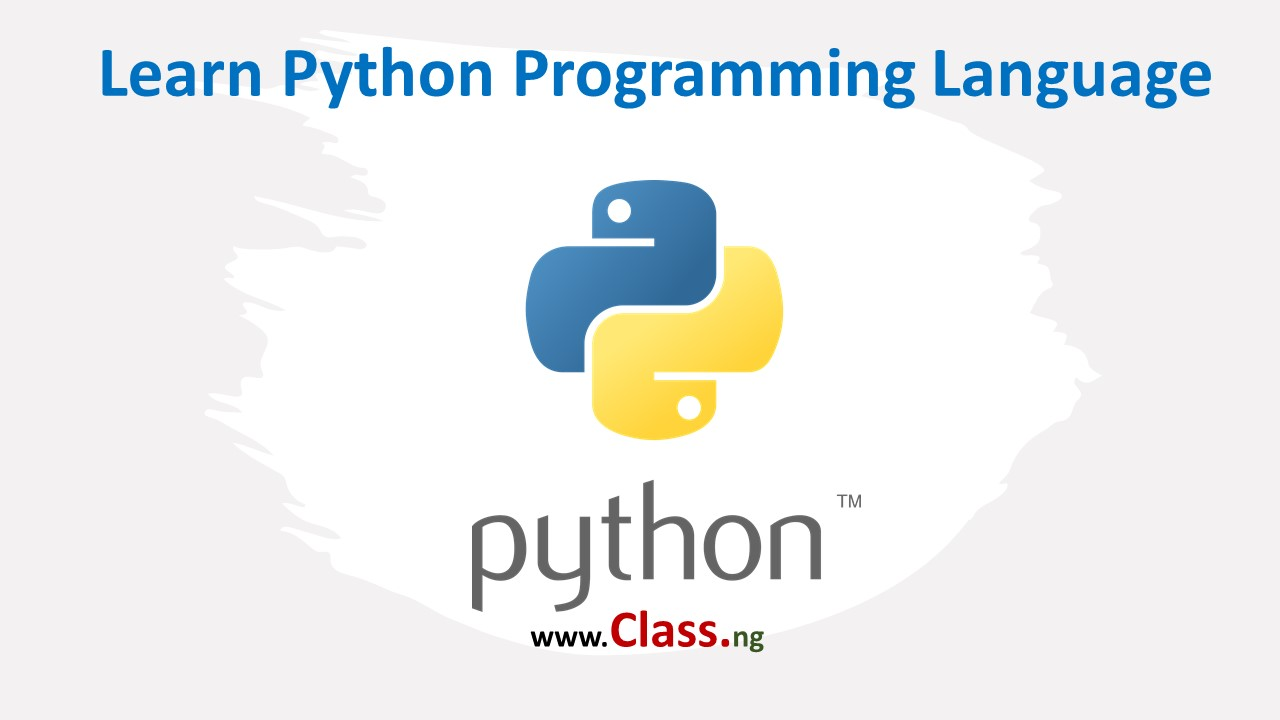-->

# Learn Python Core the Easy Way

01:35Python Language
Python is the one of today's most in-demand programming languages and the easiest to learn. Python is a high-level programming language, with applications in numerous areas, including web programming, scripting, scientific computing, and artificial intelligence! It is very popular and used by organizations such as Google, NASA, the CIA, and Disney.

In this course we will be learning Python version 3, which is the most recent major version of Python. Get your trunk ready. No time for long thing. Let's dive in!

To practice and run your codes using an online Integrated Development Environment (IDE), please click here. The online IDE will be open on another tab so you can always go to the new tab to practice. Write you codes on the left pain and click the "Run" button to run your your codes.

#### Printing text in Python

Let's start off by creating a short program that displays "Hello world!". In Python, we use the print statement to output text.

 ```print('Hello world!') ```
Output:
 ```Hello world! ```

Note that the text should be enclosed into single or double quotes. The print statement needs to be followed by parentheses, which enclose the output we want to generate. The print statement can also be used to output multiple lines of text. For Example:

 ```print('Hello world!') print('Hello world!') print('Spam and eggs...') ```
Output:
 ```Hello world! Hello world! Spam and eggs... ```

Each print statement outputs text from a new line. Python code often contains references to the comedy group Monty Python. This is why the words, "spam" and "eggs" are often used as placeholder variables in Python.

Practice makes perfect! There's no glory in practice, but without practice there can be no glory! Use the IDE link to practice and write real code. Write a program to print "Python is fun". Note that the sentence starts with a capital letter. Hint: Use print() function.

What's your output like? Drop us some Pythonista advise, suggestions or questions in the comment section.

#### Simple Operations in Python

Python has the capability of carrying out calculations. Enter a calculation directly into the print statement:

 ```print(2 + 2) print(5 + 4 - 3) ```

Output:

`4`
```6
```

The spaces around the plus and minus signs here are optional (the code would work without them), but they make it easier to read.  Python also carries out multiplication and division, using an asterisk * to indicate multiplication and a forward slash / to indicate division. Use parentheses to determine which operations are performed first.

print( 2 * (3 + 4) )
print( 10 / 2 )

14
5.0

Using a single slash to divide numbers produces a decimal (or float, as it is called in programming). We'll have more about floats in the next lesson.

Floats

Floats are used in Python to represent numbers that aren't integers (whole numbers). Some examples of numbers that are represented as floats are 0.5 and -7.8237591. They can be created directly by entering a number with a decimal point, or by using operations such as division on integers. Computers can't store floats perfectly accurately, in the same way that we can't write down the complete decimal expansion of 1/3 (0.3333333333333333...). Keep this in mind, because it often leads to infuriating bugs! As you saw previously, dividing any two integers produces a float. A float is also produced by running an operation on two floats, or on a float and an integer. A float can be added to an integer, because Python silently converts the integer to a float.

Exponentiation

Besides addition, subtraction, multiplication, and division, Python also supports exponentiation, which is the raising of one number to the power of another. This operation is performed using two asterisks. You can chain exponentiations together. In other words, you can rise a number to multiple powers. For example, 2**3**2 is same as 2**9 which equals 512.

You can also use floats in exponentiation. For example, the following code will result in the square root of 9: print(9 ** (1 / 2) ) Note that the result will be a float.

Quotient

Floor division is done using two forward slashes and is used to determine the quotient of a division (the quantity produced by the division of two numbers).

For example:

print( 20 // 6)

3

The code above will output 3, because 6 goes into 20 three times. You can also use floor division on floats.